In principle, BlochSolver sets one isochromat in one voxel. Other MRI simulators may set several or many  isochromats in one voxel. In contrast, BlochSolver divides one voxel into a number of subvoxels and sets one isochromat in one subvoxel.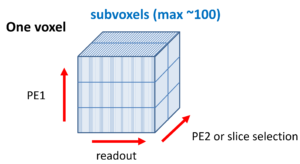Case 1. Inhomogeneous magnetic field within a voxel acquired with a gradient echo sequence : cylindrical object with different susceptibility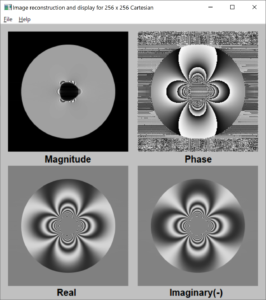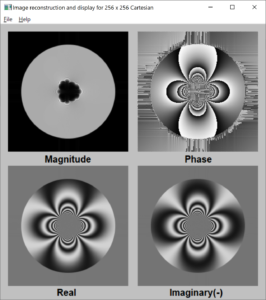subvoxel : 1×1×2: (in-plane: 1×1)      subvoxel : 2×2×2: (in-plane: 2×2)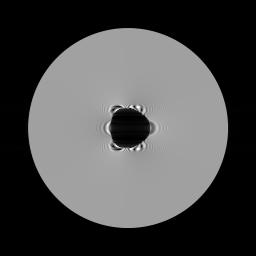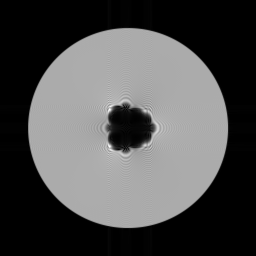absolute image (1×1)                          absolute image (2×2

The static magnetic field is applied along the horizontal direction of the MR images.

Case 2.  RF spoiled gradient echo for TR << T1, T2

2D RF spoiled gradient echo sequence, TR = 12 ms, TE = 6 ms, FA = 60°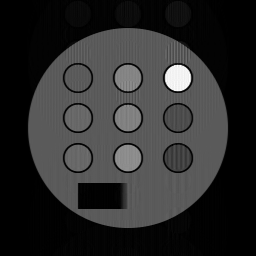subvoxel = 1×1×4 (11.223 s)  　　  subvoxel = 2×1×4 (21.944 s)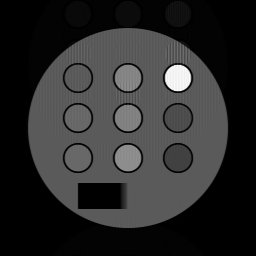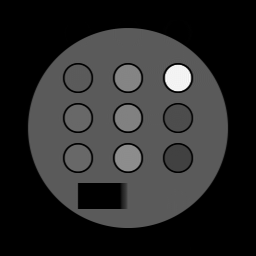subvoxel = 4×1×4 (43.421 s)   　    subvoxel = 8×1×4 (88.215 s)

The numbers in red show the calculation time using the RTX 2080Ti.

Case 3. Multiple spin echo : 16 echoes using 2π hamming windowed sinc pulse

A number of subvoxels are required in the slice direction (perpendicular to the slice plane) to describe phase accumulation in the voxel.

Image intensity vs echo time: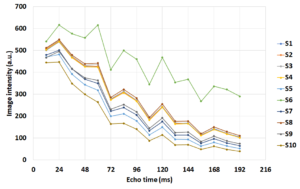The number of subvoxels = 1×1×4 (sufficient for only 4 echoes).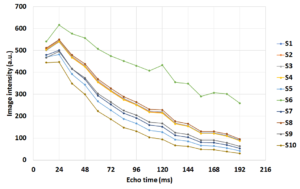The number of subvoxels = 1×1×8 (sufficient for only 8 echoes).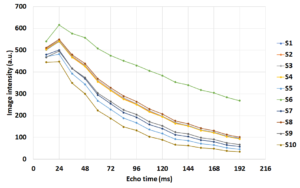The number of subvoxels = 1×1×16 (sufficient for 16 echoes).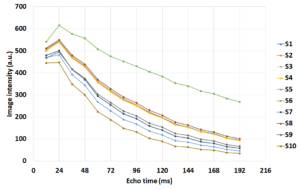The number of subvoxels = 1×1×32 (too much).